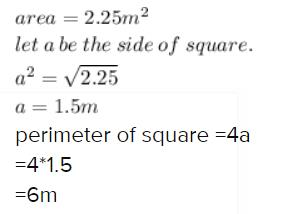Courses

# Test: Mensuration

## 10 Questions MCQ Test Mathematics (Maths) Class 6 | Test: Mensuration

Description
This mock test of Test: Mensuration for Class 6 helps you for every Class 6 entrance exam. This contains 10 Multiple Choice Questions for Class 6 Test: Mensuration (mcq) to study with solutions a complete question bank. The solved questions answers in this Test: Mensuration quiz give you a good mix of easy questions and tough questions. Class 6 students definitely take this Test: Mensuration exercise for a better result in the exam. You can find other Test: Mensuration extra questions, long questions & short questions for Class 6 on EduRev as well by searching above.
QUESTION: 1

### The length and breadth of a rectangle are 40 cm and 10 cm respectively. Its perimeter is

Solution:

We know, Perimeter of a rectangle is  2 ( l + b )

Here Length (l) = 40 cm and Breadth (b) = 10 cm

So putting the values in the above equation we have :

2 ( 40 + 10 ) = 2(50) = 100 cm

So perimeter of a rectangle is 100 cm.

QUESTION: 2

### The length of a rectangle is 150 cm. If its breadth is 1 m, then its perimeter is

Solution:

Length of rectangle=150 cm = 1.5 m
=2(1.5+1)
=2×2.5
=5.0 m

QUESTION: 3

### The area of square is 100 cm2. Its side is

Solution:
QUESTION: 4

The side of a square is 12 m. Its perimeter is

Solution:

Perimeter of square is 4 X side

thus 4 X 12 = 48 m

QUESTION: 5

If the area of a square is 64 cm2, then its perimeter is

Solution:
QUESTION: 6

If the area of a square is 2.25 m2, then its perimeter is

Solution:QUESTION: 7

The side of a square is 6 cm. If its side is doubled, then its new perimeter is

Solution:
QUESTION: 8

The area of a rectangular sheet is 500 cm2. If the length of the sheet is 25 cm, what is its width?

Solution:
QUESTION: 9

The side of a square is 8 cm. Its area is

Solution:
QUESTION: 10

The area of a rectangle is 96 cm2. If the breadth of the rectangle is 8 cm, find its perimeter.

Solution:

Area of rectangle = L×B
96 = L×8
L = 96/8
Length of rectangle = 12cm
Perimeter of rectangle = 2×(l+b)
= 2× (12+8)
= 2×20
Perimeter of rectangle =40 cm